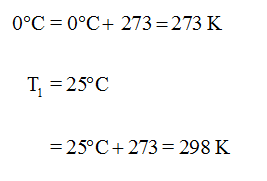# A sample of CH4 in a cylinder with a moveable piston has a 4100 torr pressure at a temperature of 25 °C when the volume is 5100 mL. Calculate the volume (mL) of gas present if the temperature and pressure are changed to standard temperature (273 K) and pressure (760 torr).

Question
1 views

A sample of CH4 in a cylinder with a moveable piston has a 4100 torr pressure at a temperature of 25 °C when the volume is 5100 mL. Calculate the volume (mL) of gas present if the temperature and pressure are changed to standard temperature (273 K) and pressure (760 torr).

check_circle

Step 1

Unit of temperature is converted as follows,...

### Want to see the full answer?

See Solution

#### Want to see this answer and more?

Solutions are written by subject experts who are available 24/7. Questions are typically answered within 1 hour.*

See Solution
*Response times may vary by subject and question.
Tagged in

### Chemistry